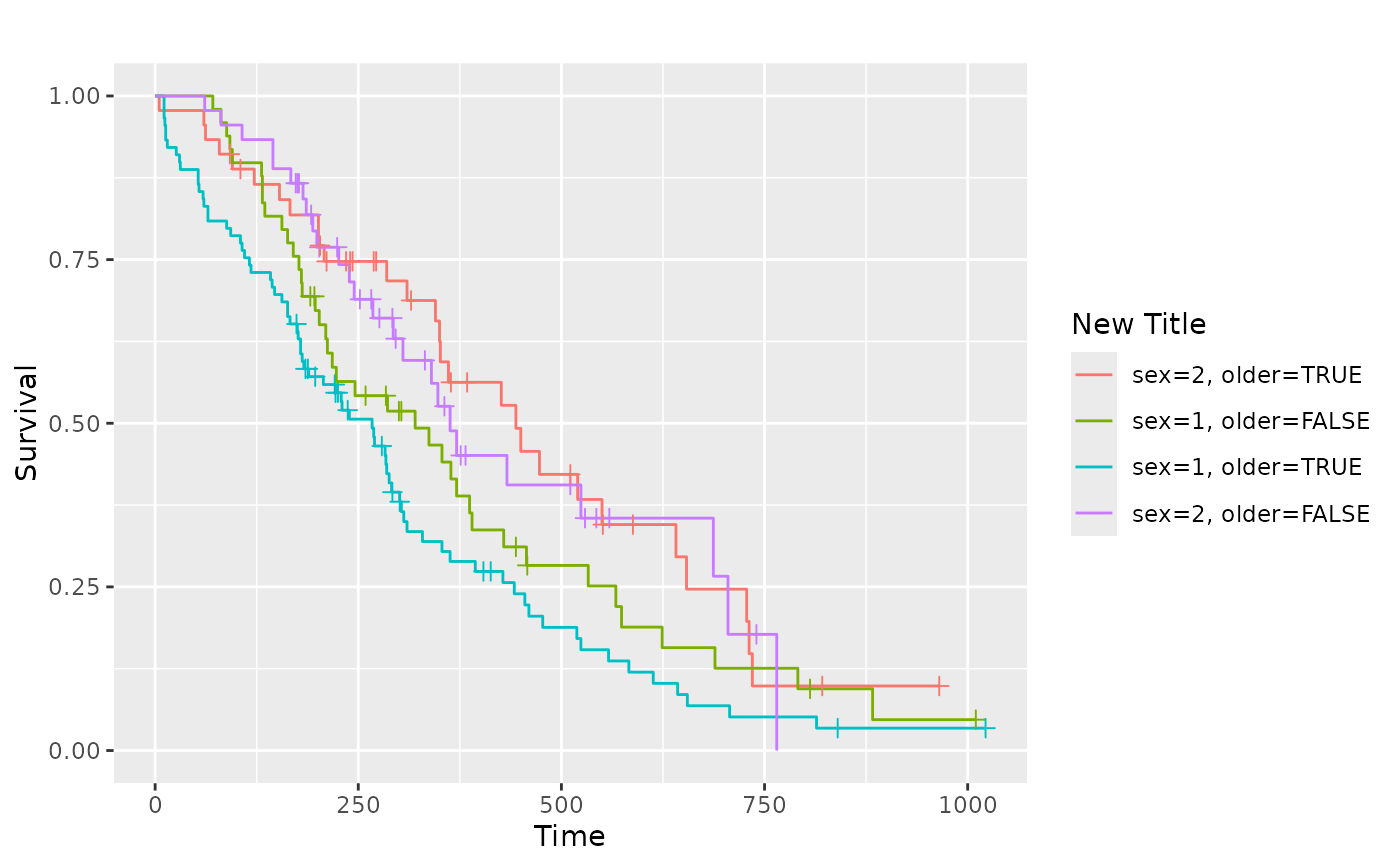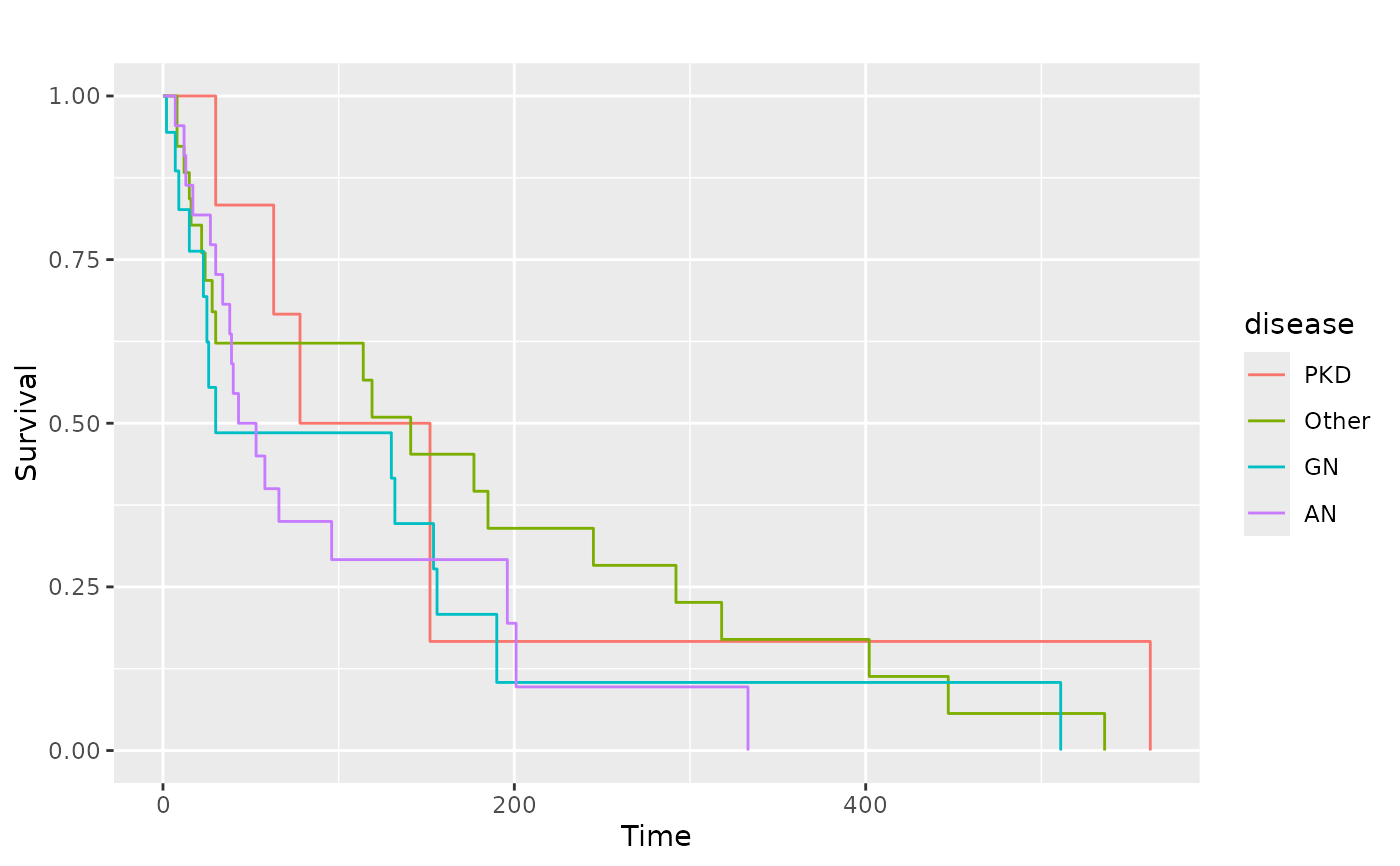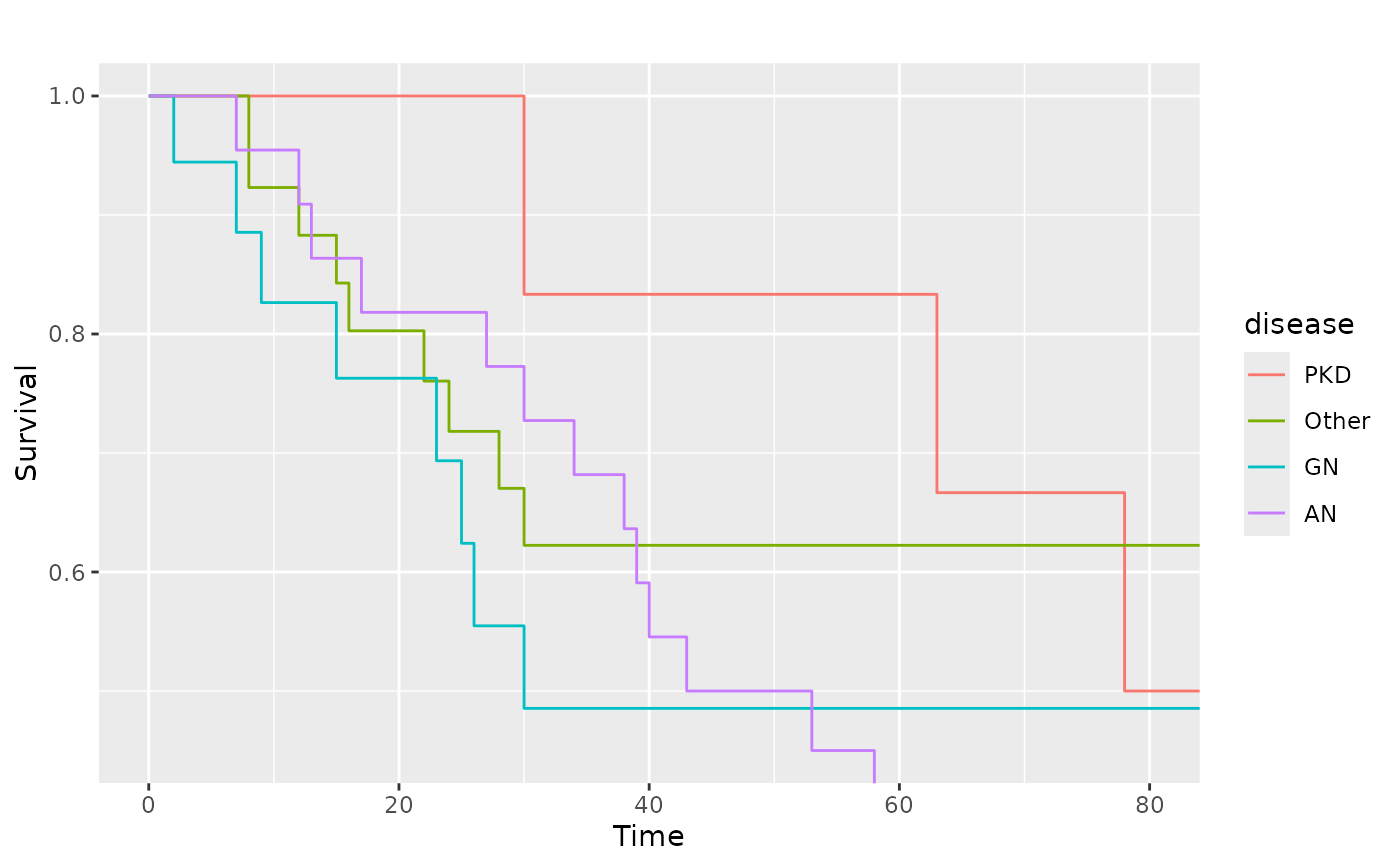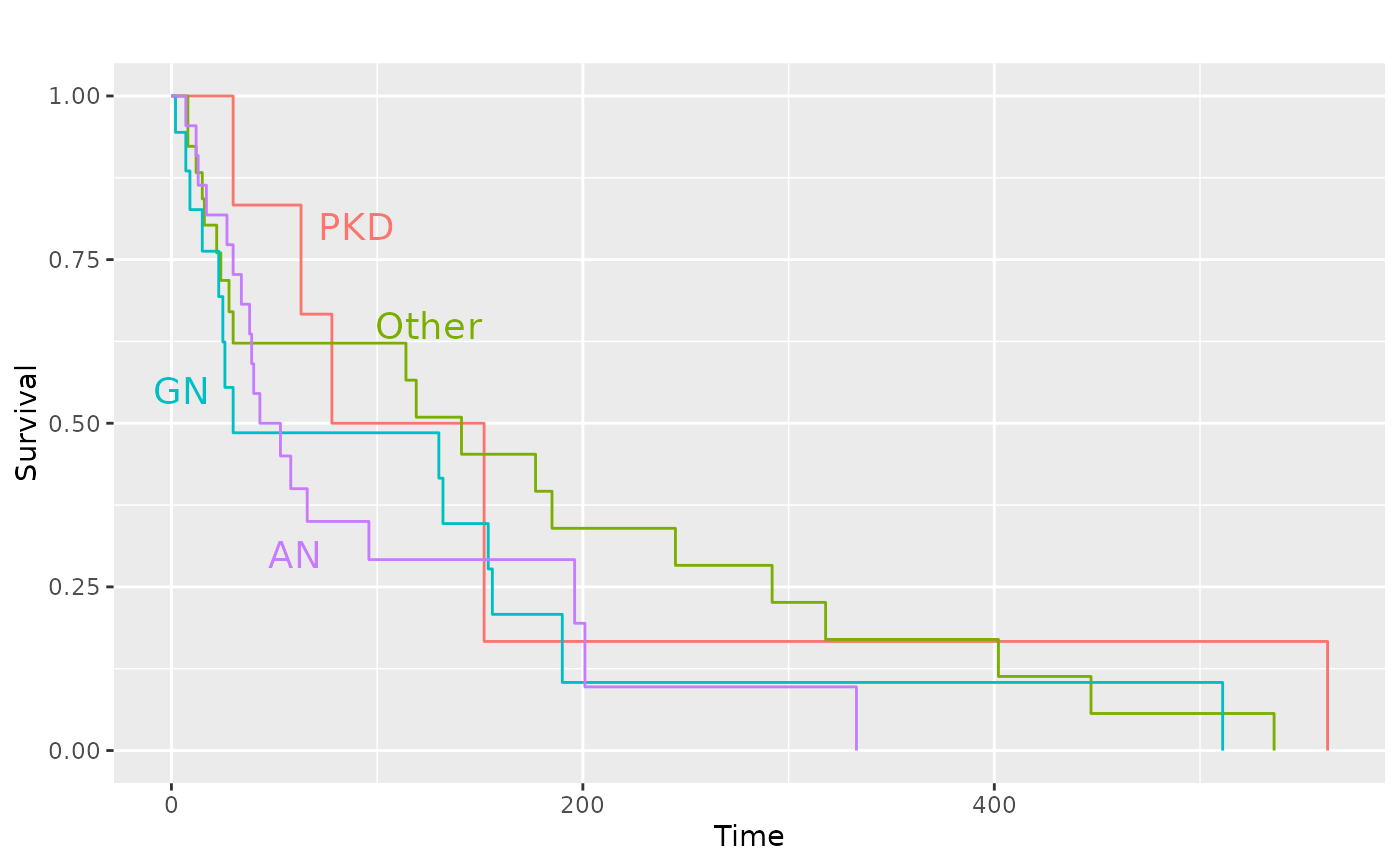This function produces Kaplan-Meier plots using ggplot2. As a first argument it needs a survfit object, created by the survival package. Default settings differ for single stratum and multiple strata objects.

ggsurv(
s,
CI = "def",
plot.cens = TRUE,
surv.col = "gg.def",
cens.col = "gg.def",
lty.est = 1,
lty.ci = 2,
size.est = 0.5,
size.ci = size.est,
cens.size = 2,
cens.shape = 3,
back.white = FALSE,
xlab = "Time",
ylab = "Survival",
main = "",
order.legend = TRUE
)

## Arguments

s an object of class survfit should a confidence interval be plotted? Defaults to TRUE for single stratum objects and FALSE for multiple strata objects. mark the censored observations? colour of the survival estimate. Defaults to black for one stratum, and to the default ggplot2 colours for multiple strata. Length of vector with colour names should be either 1 or equal to the number of strata. colour of the points that mark censored observations. linetype of the survival curve(s). Vector length should be either 1 or equal to the number of strata. linetype of the bounds that mark the 95% CI. line width of the survival curve line width of the 95% CI point size of the censoring points shape of the points that mark censored observations. if TRUE the background will not be the default grey of ggplot2 but will be white with borders around the plot. the label of the x-axis. the label of the y-axis. the plot label. boolean to determine if the legend display should be ordered by final survival time

## Value

An object of class ggplot

Edwin Thoen

## Examples

# Small function to display plots only if it's interactive
p_ <- GGally::print_if_interactive

if (require(survival) && require(scales)) {
data(lung, package = "survival")
sf.lung <- survival::survfit(Surv(time, status) ~ 1, data = lung)
p_(ggsurv(sf.lung))

# Multiple strata examples
sf.sex <- survival::survfit(Surv(time, status) ~ sex, data = lung)
pl.sex <- ggsurv(sf.sex)
p_(pl.sex)

# Adjusting the legend of the ggsurv fit
p_(pl.sex +
ggplot2::guides(linetype = "none") +
ggplot2::scale_colour_discrete(
name   = 'Sex',
breaks = c(1,2),
labels = c('Male', 'Female')
))

# Multiple factors
lung2 <- plyr::mutate(lung, older = as.factor(age > 60))
sf.sex2 <- survival::survfit(Surv(time, status) ~ sex + older, data = lung2)
pl.sex2 <- ggsurv(sf.sex2)
p_(pl.sex2)

# Change legend title
p_(pl.sex2 + labs(color = "New Title", linetype = "New Title"))

# We can still adjust the plot after fitting
data(kidney, package = "survival")
sf.kid <- survival::survfit(Surv(time, status) ~ disease, data = kidney)
pl.kid <- ggsurv(sf.kid, plot.cens = FALSE)
p_(pl.kid)

# Zoom in to first 80 days
p_(pl.kid + ggplot2::coord_cartesian(xlim = c(0, 80), ylim = c(0.45, 1)))

# Add the diseases names to the plot and remove legend
p_(pl.kid +
ggplot2::annotate(
"text",
label  = c("PKD", "Other", "GN", "AN"),
x      = c(90, 125, 5, 60),
y      = c(0.8, 0.65, 0.55, 0.30),
size   = 5,
colour = scales::hue_pal(
h         = c(0, 360) + 15,
c         = 100,
l         = 65,
h.start   = 0,
direction = 1
)(4)
) +
ggplot2::guides(color = "none", linetype = "none"))
}
#> Loading required package: scales#> Warning: data set ‘lung’ not found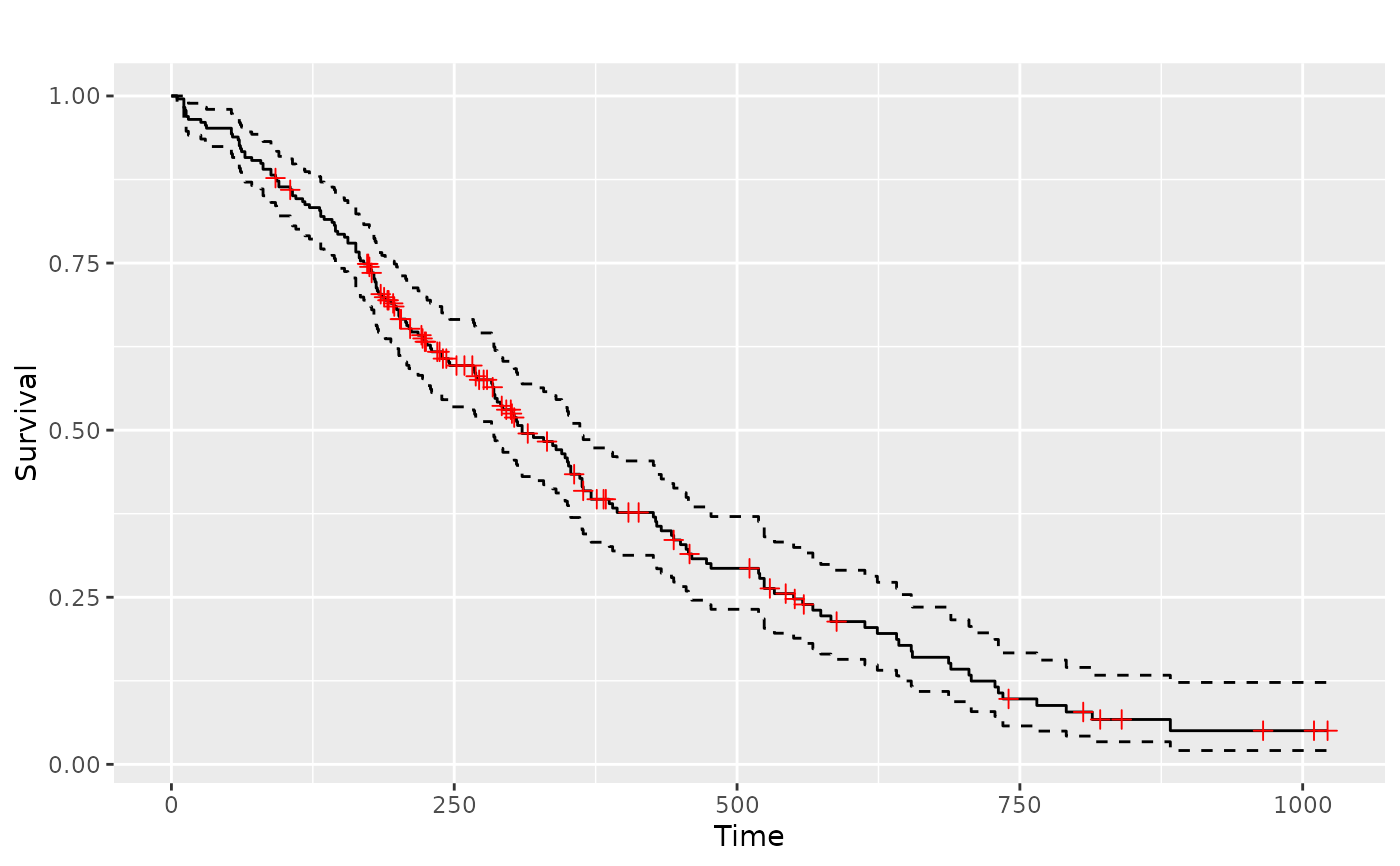#> Scale for 'colour' is already present. Adding another scale for 'colour',
#> which will replace the existing scale.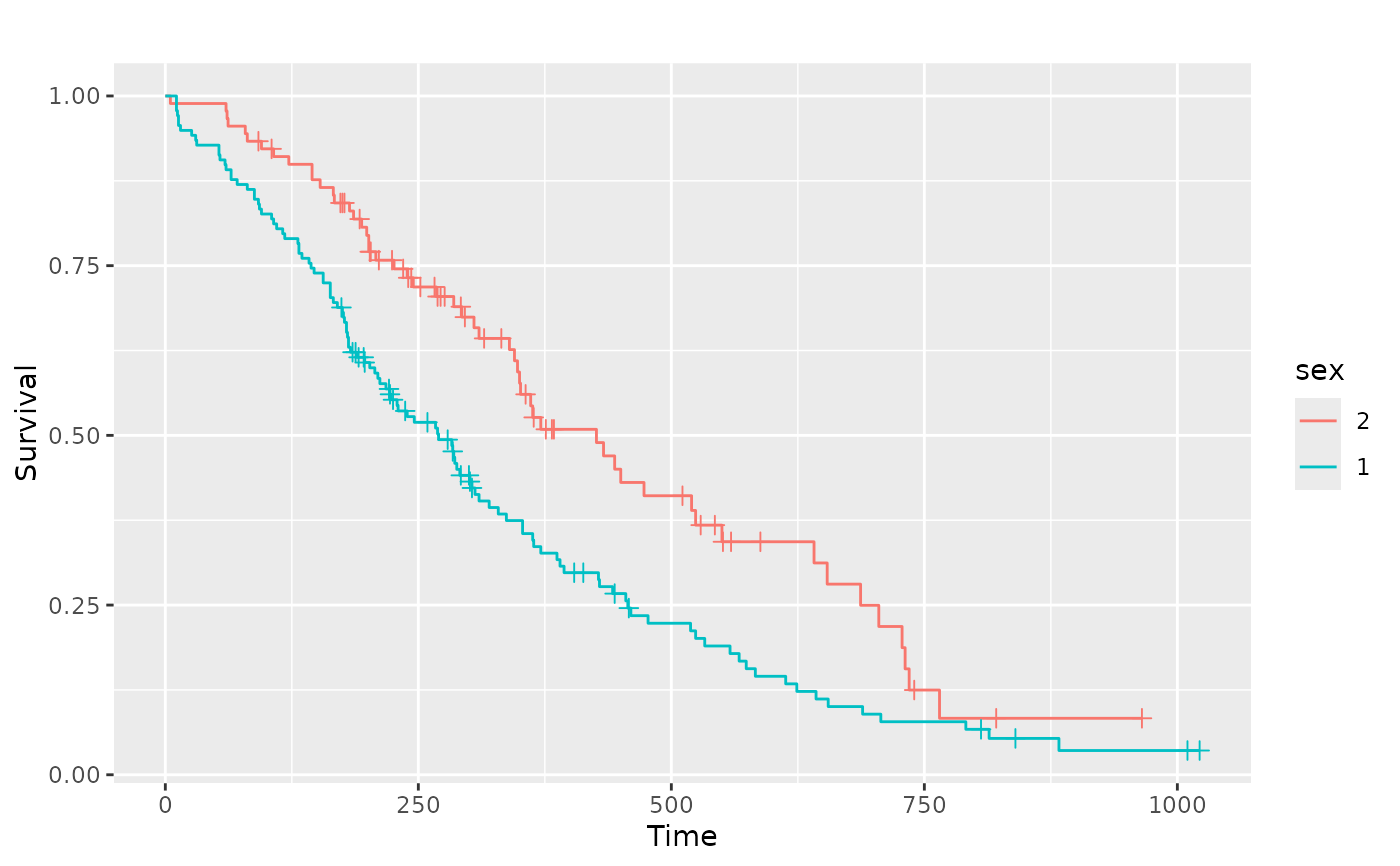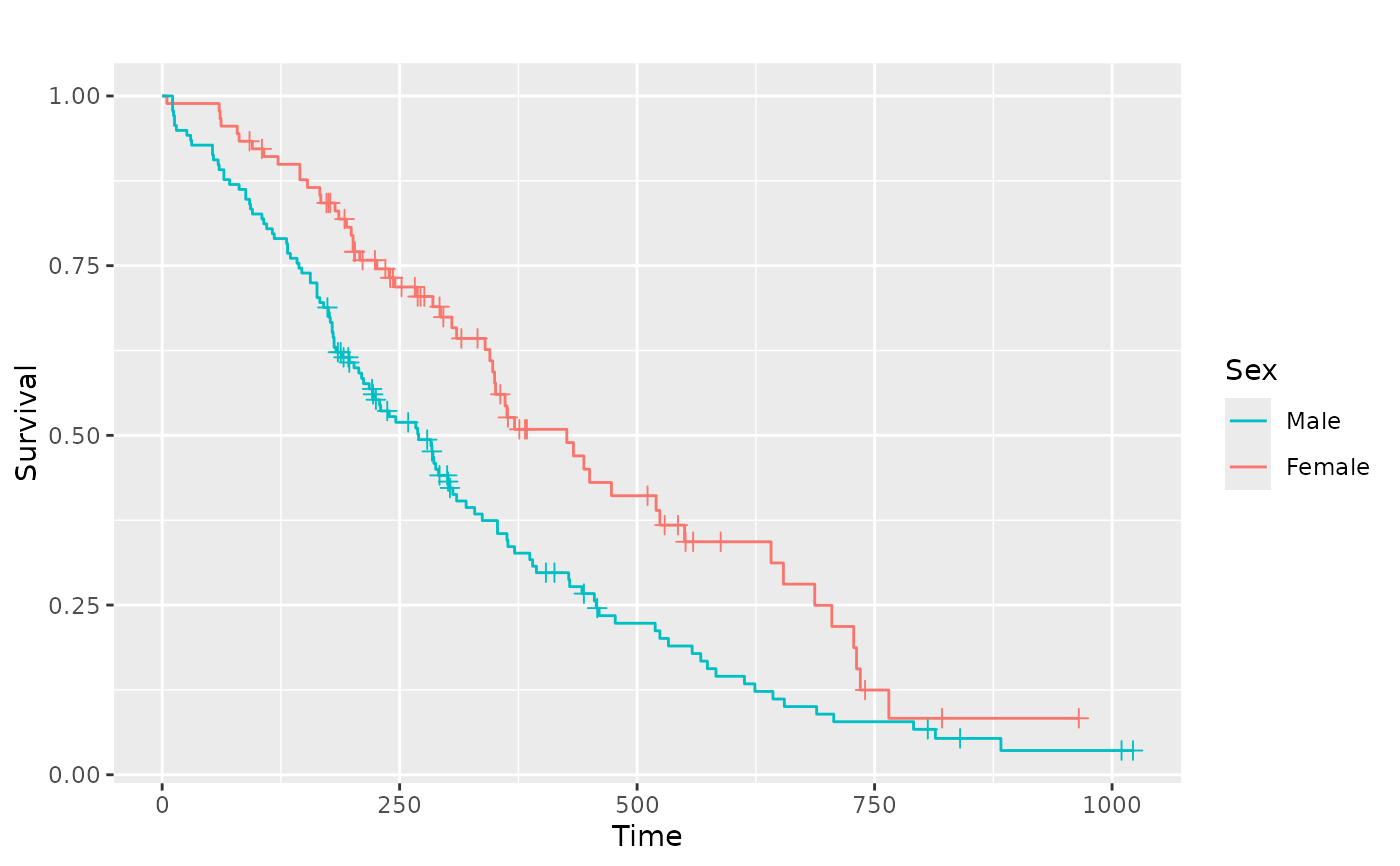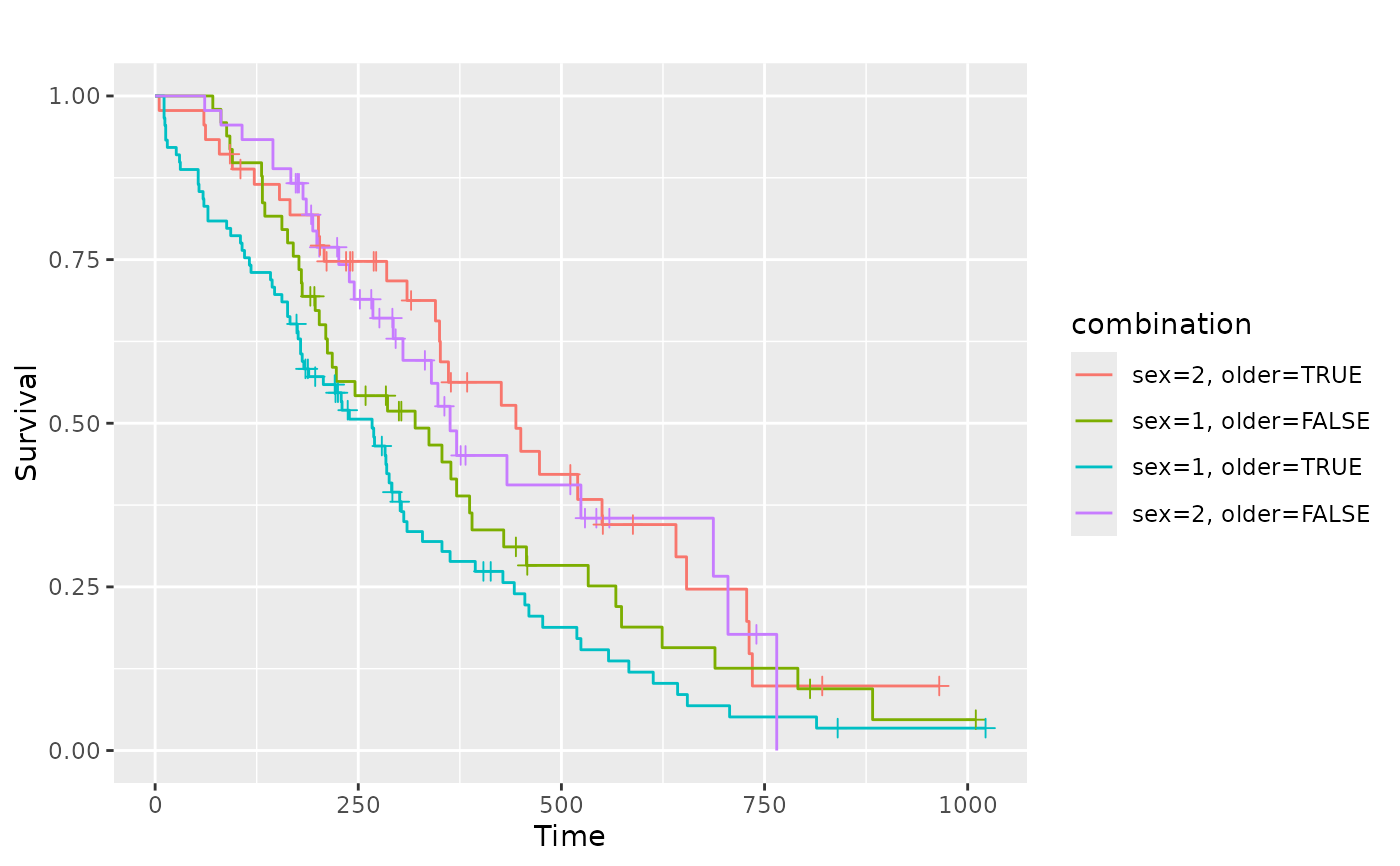#> Warning: data set ‘kidney’ not found